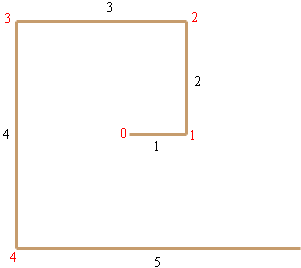SEARCH HOMEMath Central Quandaries & QueriesQuestion from Pinar, a parent: I am trying to help my daughter with very challenging maths questions which sometimes I get stuck If someone help me with one of them I would appreciate it. Jane is making a spiral out of wire. She bends the wire after 1 cm, then bends the wire after 2cm, then 3cm and continues in this manner. After 4 bends she used 15 cm of wire For each bend how many cm wire was used? After 6 bends how many cm wire will she have used? How many bends will she have made if she uses 66 cm of wire? I would appreciate if somebody would help me with this. Thank you! PinarHi Pinar,

Here is my picture of the wire after 4 bends.I have labeled the length of each section of wire and numbered the bends in red, calling the start of the wire bend zero. The length of wire used so far is $1 + 2 + 3 + 4 + 5 = 15$ centimeters.

In the diagram notice that

the length of the segment after bend 0 is 1 cm
the length of the segment after bend 1 is 2 cm
the length of the segment after bend 2 is 3 cm
the length of the segment after bend 3 is 4 cm
the length of the segment after bend 4 is 5 cm.

Following this pattern

the length of the segment after bend 6 is 7.

Hence the length of wire used after 6 bends is $1 + 2 + 3 + 4 + 5 + 6 + 7$ cm.

What is the length of wire used after 7 bends? After 8 bends? How far do you need to go to get a length of 66 cm.

It would be helpful if there were a nice formula for $1 + 2 + 3 + \cdot \cdot \cdot + n$ where $n$ is an integer. There is but it isn't necessary for numbers as small as you have in this problem.

PennyMath Central is supported by the University of Regina and The Pacific Institute for the Mathematical Sciences.